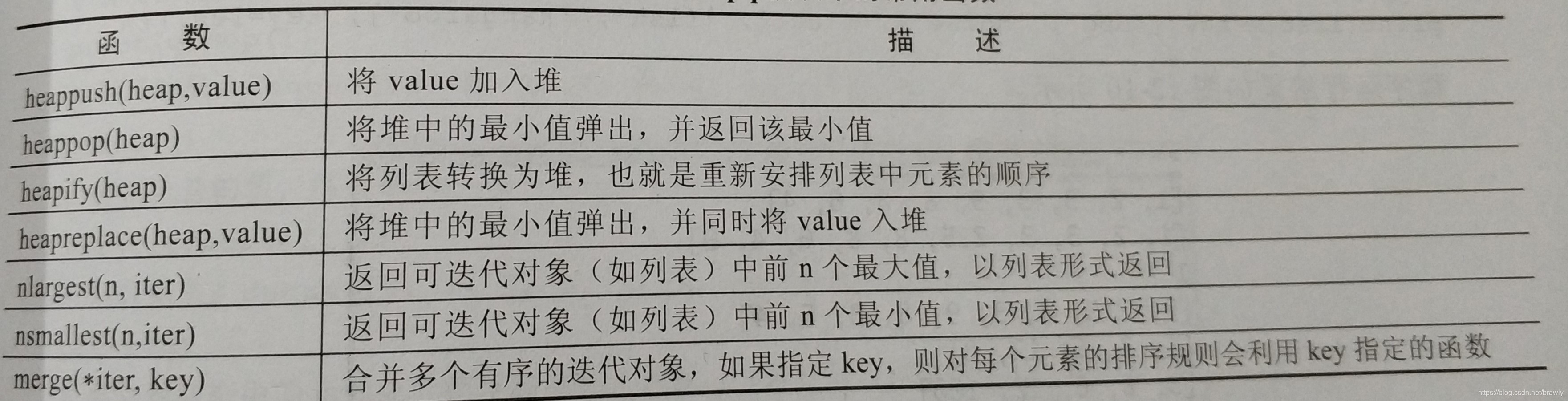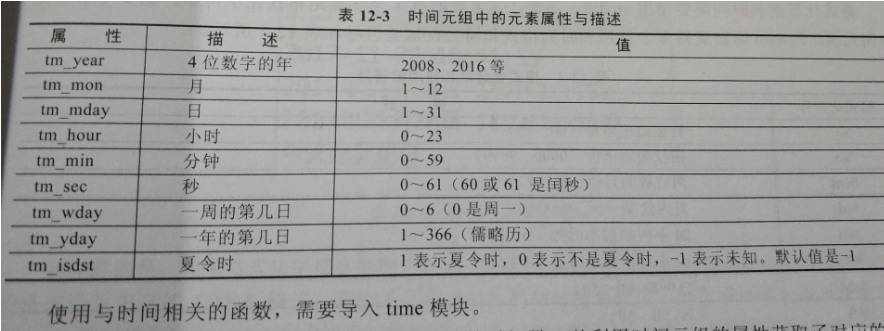# Python中的常用模块（下）

+关注继续查看

## 3.2 堆# 堆（Heap）

# heapq

from heapq import *
from random import *

data = [1,2,3,4,5,6,7,8,9]
heap = []   # 定义一个堆，其实堆就是一个列表，只是通过heapq模块中的函数利用堆算法来改变列表中的元素而已。
for n in data:
value = choice(data)  # 利用choice函数（随机random模块中的）从data列表中随机选择9个数（可能有重复的数）
heappush(heap,value)  # 利用heappush函数将value添加进堆
print(heap)  # 运行结果：[1, 1, 6, 1, 4, 9, 9, 8, 7]

heappush(heap,30)
heappush(heap,45)
print(heap)   # 运行结果：[1, 1, 6, 1, 4, 9, 9, 8, 7, 30, 45]

print(heappop(heap))   # heappop()将堆中的最小值弹出，并返回最小值。运行结果：1

data1 = [6,3,1,12,9]  # 定义一个列表
heapify(data1)    # heapify() 将列表转换为堆，也就是重新安排列表中元素的顺序，直接修改了（data1）
print(data1)   # 运行结果：[1, 3, 6, 12, 9]

print(heapreplace(data1, 123))  # 弹出data1的最小值，并将123添加进堆。  有返回值，运行结果：1
print(data1)   # 运行结果：[3, 9, 6, 12, 123]

print(nlargest(1,data1))  # 得到data1中的最大值，以列表形式返回。运行结果：
print(nlargest(2,data1))  # 得到data1中最大的前2个值，运行结果：[123, 12]

print(nsmallest(1,data1))  # 得到data1中的最小值，以列表形式返回。运行结果：
print(nsmallest(2,data1))  # 得到data1中的最小值，以列表形式返回。运行结果：[3, 6]

print(list(merge([1,3,456,7],[0,1,-5,6],[1,7,4],[],)))  # 运行结果：[0, 1, 1, -5, 1, 3, 6, 7, 4, 67, 456, 7]

print(list(merge(['dog','horse'],['cat','fish','kangaroo'],key=len))) # 运行结果：['dog', 'cat', 'fish', 'horse', 'kangaroo']


## 3.3 双端队列

# 双端队列

from collections import deque

# 将列表转换为双端队列
q = deque(range(10))
print(q)    # 运行结果： deque([0, 1, 2, 3, 4, 5, 6, 7, 8, 9])

# 将123追加到双端队列q的队尾
q.append(123)
q.append(-32)
print(q)    # 运行结果：deque([0, 1, 2, 3, 4, 5, 6, 7, 8, 9, 123, -32])

# 将30添加到q的队首
q.appendleft(30)
print(q)   # 运行结果：deque([30, 0, 1, 2, 3, 4, 5, 6, 7, 8, 9, 123, -32])

# 弹出队尾的值
print(q.pop())  # 运行结果 -32
# 弹出队首的值
print(q.popleft())   # 运行结果： 30

print(q)   # 运行结果 deque([0, 1, 2, 3, 4, 5, 6, 7, 8, 9, 123])

# 将q向左循环移动2个位置
q.rotate(-2)
print(q)  # 运行结果 deque([2, 3, 4, 5, 6, 7, 8, 9, 123, 0, 1])

# 向右循环移动4个位置
q.rotate(4)
print(q)   # 运行结果 deque([9, 123, 0, 1, 2, 3, 4, 5, 6, 7, 8])

# 创建一个双端队列q1
q1 = deque(['a','b'])
q.extend(q1)  # 将q1添加到队列q的后面
print(q)   # 运行结果 deque([9, 123, 0, 1, 2, 3, 4, 5, 6, 7, 8, 'a', 'b'])

q.extendleft(q1)  # 将q1添加到队列q的前面
print(q)   # 运行结果  deque(['b', 'a', 9, 123, 0, 1, 2, 3, 4, 5, 6, 7, 8, 'a', 'b'])


# 4、时间、日期与日历（time模块）

Python提供的time、datetime和calendar模块可以用于格式化日期和时间。时间间隔是以秒为单位的浮点数。每个时间戳从1970年1月1日午夜（历元）到现在经过的时间来表示。

Python语言的time模块下有很多函数可以转换常见日期格式。例如：函数time用于获取当前时间戳，

import time
ticks=time.time()
print("当前的时间戳：", ticks)
# 运行结果          当前的时间戳： 1575013913.8061256


ticks变量的值是一个浮点数，这个浮点数就是从运行程序那一刻的时间点到1970年1月1日午夜之间的秒数。

## 4.1 时间元组# 时间元组（元组里面的值不可更改，元组没有属性）
import time
# 获取当前时间
localtime = time.localtime(time.time())
# 运行结果 time.struct_time(tm_year=2019, tm_mon=11, tm_mday=29, tm_hour=16, tm_min=17, tm_sec=12, tm_wday=4, tm_yday=333, tm_isdst=0)
print(localtime)  # localtime是一个对象

print(type(localtime))   # 运行结果 <class 'time.struct_time'>
# 创建struct_time这个对象时，通过元组来指定每个时间，之后会把对应的值放在每个分量里面
st = time.struct_time((1,2,3,4,5,6,7,8,9))
# 运行结果 time.struct_time(tm_year=1, tm_mon=2, tm_mday=3, tm_hour=4, tm_min=5, tm_sec=6, tm_wday=7, tm_yday=8, tm_isdst=9)
print(st)

print('年','=',localtime.tm_year)    # 运行结果   年 = 2019
print('月','=',localtime.tm_mon)     # 运行结果   月 = 11
print('日','=',localtime.tm_mday)    # 运行结果   日 = 29
print('一年的第%d天' % localtime)  # 运行结果   一年的第333天

# 获取一个可读的时间
localtime = time.asctime(localtime)
print(localtime)          # Fri Nov 29 16:32:47 2019


## 4.2 格式化日期和时间

strftime函数，参数1：格式化字符串 参数2：时间元组

Python语言中，所有用于格式化日期和时间的符号都以百分号（%）开头，如 %Y-%m-%d 会将日期格式化为如 “2019-11-26”。

%y 两位数的年份表示（00-99）
%Y 四位数的年份表示（000-9999）
%m 月份（01-12）
%d 月内中的一天（0-31）
%H 24小时制小时数（0-23）
%I 12小时制小时数（01-12）
%M 分钟数（00=59）
%S 秒（00-59）
%a 本地简化星期名称
%A 本地完整星期名称
%b 本地简化的月份名称
%B 本地完整的月份名称
%c 本地相应的日期表示和时间表示
%j 年内的一天（001-366）
%p 本地A.M.或P.M.的等价符
%U 一年中的星期数（00-53）星期天为星期的开始
%w 星期（0-6），星期天为星期的开始
%W 一年中的星期数（00-53）星期一为星期的开始
%x 本地相应的日期表示
%X 本地相应的时间表示
%Z 当前时区的名称
%% %号本身

# 格式化日期和时间

# strftime函数，参数1：格式化字符串   参数2：时间元组

import time
import locale

# 2018-12-23 18:34:22
print(time.strftime('%Y-%m-%d %H:%M:%S',time.localtime()))  # 运行结果 2019-11-29 17:23:00

# 设置日期和时间为中文UTF-8格式，格式化字符串中支持中文
locale.setlocale(locale.LC_ALL, 'zh_CN.UTF-8')

# 运行结果 2019年11月29日 17时23分0秒
print(time.strftime('%Y年%m月%d日 %H时%M分%S秒',time.localtime()))
# 输出星期的完整名称
# 今天是星期五
print(time.strftime('今天是%A',time.localtime()))


## 4.3 时间戳的增量

import time
time1 = time.time()    # 获取当前的时间戳

time2 = time1 + 60     # 当前时间戳 往后 移1分钟

print(time2)       # 运行结果 1575093743.3013074

time3 = time1 - 60*60  # 当前时间戳 往前 移1个小时

time3= time.localtime(time3)   # 将时间戳转化为时间元组
print(time3)   # 运行结果 time.struct_time(tm_year=2019, tm_mon=11, tm_mday=30, tm_hour=13, tm_min=1, tm_sec=23, tm_wday=5, tm_yday=334, tm_isdst=0)

# 运行结果 2019-11-30 13:01:23
print(time.strftime('%Y-%m-%d %H:%M:%S', time3))


## 4.4 计算日期和时间的差值

datetime模块中的datetime类允许计算两个日期的差值，可以得到任意两个日期之间的天数以及剩余的秒数。通过datetime模块的timedelta函数还可以得到一个时间增量，如 timedetla（hours=2）可得到往后延两个小时的时间增量。

# 计算日期和时间的差

# timedetla

import datetime

# 定义第一个日期
d1 = datetime.datetime(2017,4,12)
# 定义第二个日期
d2 = datetime.datetime(2018,12,25)
# 计算这两个日期之间的天数，运行结果 622
print((d2-d1).days)

# 定义第1个带时间的日期
d1 = datetime.datetime(2017,4,12,10,10,10)
# 定义第2个带时间的日期
d2 = datetime.datetime(2018,12,25,10,10,40)
# 输出两个日期的差，运行结果  622 days, 0:00:30
print(d2 - d1)

# 计算两个日期相差的秒数（刨除整数天），运行结果 30
print((d2 - d1).seconds)

# 获取当前的时间（datetime类型）
d1 = datetime.datetime.now()
# 将当前时间往后延 10 小时
d2 = d1 + datetime.timedelta(hours=10)
# 运行结果 2019-12-01 00:28:07.977654
print(d2)
d2 = d1 + datetime.timedelta(hours=-10)
# 运行结果 2019-11-30 04:28:07.977654
print(d2)


## 4.5 获取某月和某年的日历

# 获取某月和某年的日历

import calendar
import locale

# 返回2018年1月份日历（日历是英文的）
cal = calendar.month(2018,1)
print(cal)

# 恢复默认的日期格式(英文)，否则显示12个月的日历，位置会窜
# locale.setlocale(locale.LC_ALL, '')

# 输出2018年一整年的日历(共12个月)
print(calendar.calendar(2018))



# 5.随机数（random模块）

# 随机数

'''
randint(m,n):用于产生m到n之间的随机整数，包括m和n。

random()：用于产生0到1之间的随机浮点数，包括0，但不包括1。

uniform(m,n)：用于产生m到n之间的随机浮点数，m和n可以是浮点数，包括m和n。

randrange(m,n,step)：在一个递增的序列中随机选择一个整数。其中step是步长。

choice(seq)：从seq指定的序列中随机选择一个元素值。seq指定的列表元素可以是任意类型的值。

sample(seq,k)：从seq指定的序列中随机选取k个元素，然后生成一个新的序列。

shuffle(seq)：把seq指定的序列中元素的顺序打乱，该函数直接修改原有的序列。
'''

import random
# 产生1到100之间的随机整数
print(random.randint(1,100))     # 运行结果 19

# 产生0到1之间的随机数
print(random.random())            # 运行结果 0.12475554094811458
# 从[1,4,7,10,13,16,19]随机选一个数
print(random.randrange(1,20,3))    # 运行结果 19

# 产生一个从1到100.5的随机浮点数
print(random.uniform(1,100.5))     # 运行结果 46.42341579462214

# 从intList列表中随机选一个元素值
intList = [1,2,5,7,76,10,4,8]
print(random.choice(intList))      # 运行结果 1

# 从intList列表中随机选3个元素值，并生成一个新的序列
newList = random.sample(intList,3)
print(newList)                      # 运行结果  [2, 8, 7]

# 随机排列intList列表中的元素值，该函数直接改变了intList列表(洗牌)
random.shuffle(intList)
print(intList)                     # 运行结果 [1, 2, 5, 8, 10, 4, 76, 7]



# 6.数学（math模块）

# 数学函数

import math
print('圆周率','=',math.pi)    # 运行结果   圆周率 = 3.141592653589793
print('自然常数','=',math.e)   # 运行结果   自然常数 = 2.718281828459045

# 取绝对值
print(math.fabs(-1.2))     #  1.2

# 向上取整
print(math.ceil(1.3))      # 2

# 向下取整
print(math.floor(1.8))     # 1

# 计算2的10次方
print(math.pow(2,10))     # 1024.0

# 计算8的平方根
print(math.sqrt(8))     # 2.8284271247461903

# 计算 Π/2 的正弦
print(math.sin(math.pi/2))   # 1.0

# 计算 Π 的余弦
print(math.cos(math.pi))    #   -1.0

# 计算 Π/4 的正切
print(math.tan(math.pi/4))   # 0.999999999999999910 Python之异常/模块/包

5 0python 模块与包
python 的包就是文件夹，包中还可以有子包，也就是子文件夹。 python 的模块就是一个个.py 文件，定义了各种功能接口。将模块以文件夹的形式进行分组管理。
13 0Python 之设计模式、异常处理、模块与包、文件操作及编码
Python 之设计模式、异常处理、模块与包、文件操作及编码
32 015、python模块和包

1221 0Python模块与包
1284 0python中常用到的模块和包名称
1398 0python之 模块与包
951 0Python之异常处理、模块与包
938 0【循序渐进学Python】10.模块和包
954 0+关注
196

0